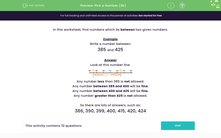# Identify Numbers in the Range 600-999

In this worksheet, students will write a number between two given numbers.Key stage:  KS 2

Curriculum topic:   Number: Number and Place Value

Curriculum subtopic:   Order/Compare Numbers to 1000

Difficulty level:#### Worksheet Overview

In this activity, we will be learning about numbers that lie between two given numbers.

Let's think about numbers which lie between 625 and 685

Sometimes it is useful to imagine the numbers on a number line, like this.This number line helps us to imagine some of the numbers which will fall between 625 and 685.

Ben has written a set of numbers between 625 and 685, but he has got one wrong.

Can you spot it?

624, 626, 658, 684

Ben has written the number 624. This is actually smaller than 625, so should not be included in the set.

Let's try an example.

Example

Which of the following numbers does not fall between 710 and 735?

712, 702, 720, 729, 733.

If we look carefully at the numbers, 712, 720, 729 and 733 all fall between 710 and 735.

702 is the odd one out. It is too small to be in this set.

Now it's your turn to try some questions like this.### What is EdPlace?

We're your National Curriculum aligned online education content provider helping each child succeed in English, maths and science from year 1 to GCSE. With an EdPlace account you’ll be able to track and measure progress, helping each child achieve their best. We build confidence and attainment by personalising each child’s learning at a level that suits them.

Get started